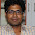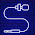Friday, July 23, 2010

Means Ends Analysis.

MEANS - ENDS ANALYSIS:-

Most of the search strategies either reason forward of backward however, often a mixture o the two directions is appropriate. Such mixed strategy would make it possible to solve the major parts of problem first and solve the smaller problems the arise when combining them together. Such a technique is called "Means - Ends Analysis".

The means -ends analysis process centers around finding the difference between current state and goal state. The problem space of means - ends analysis has an initial state and one or more goal state, a set of operate with a set of preconditions their application and difference functions that computes the difference between two state a(i) and s(j). A problem is solved using means - ends analysis by

1. Computing the current state s1 to a goal state s2 and computing their difference D12.

2. Satisfy the preconditions for some recommended operator op is selected, then to reduce the difference D12.

3. The operator OP is applied if possible. If not the current state is solved a goal is created and means- ends analysis is applied recursively to reduce the sub goal.

4. If the sub goal is solved state is restored and work resumed on the original problem.

( the first AI program to use means - ends analysis was the GPS General problem solver)

means- ends analysis I useful for many human planning activities. Consider the example of planing for an office worker. Suppose we have a different table of three rules:

1. If in out current state we are hungry , and in our goal state we are not hungry , then either the "visit hotel" or "visit Canteen " operator is recommended.

2. If our current state we do not have money , and if in your goal state we have money, then the "Visit our bank" operator or the "Visit secretary" operator is recommended.

3. If our current state we do not know where something is , need in our goal state we do know, then either the "visit office enquiry" , "visit secretary" or "visit co worker " operator is recommended.

1.2.